# DAV Class 4 Maths Chapter 13 Worksheet 2 Solutions

The DAV Maths Class 4 Solutions and DAV Class 4 Maths Chapter 13 Worksheet 2 Solutions of Volume offer comprehensive answers to textbook questions.

## DAV Class 4 Maths Ch 13 WS 2 Solutions

Question 1.
Find the volume of the following solids in terms of the unit of volume (cubes).

(a)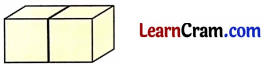Solution:
2 cubes

(b)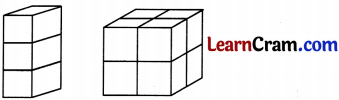Solution:
6 cubes

(c)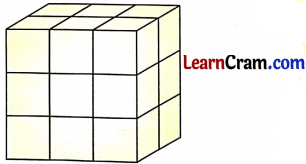Solution:
18 cubes

(d)Solution:
45 cubesQuestion 2.
Which of the following is the unit to measure volume?
(a)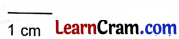(b)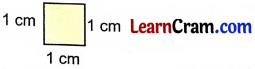(c)Solution:

(c)### DAV Class 4 Maths Chapter 13 Worksheet 2 Notes

• A cube is the best unit of measurement for volume.
• Measure the volume of following solid in terms of unit of volume (cubes).